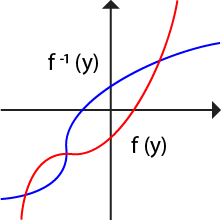Uh Oh! It seems you’re using an Ad blocker!

Since we’ve struggled a lot to makes online calculations for you, we are appealing to you to grant us by disabling the Ad blocker for this domain.# Inverse Function Calculator

Enter a function: y = f(x) =

Table of Content
 1 Inverse Function Graph: 2 How to Calculate Inverse Function (Step-Wise): 3 What is the difference between reciprocal & inverse function? 4 How inverse function used for temperature conversion? 5 What is the inverse of 1/ x?
Get The Widget!

Add Inverse Function Calculator to your website to get the ease of using this calculator directly. Feel hassle-free to account this widget as it is 100% free, simple to use, and you can add it on multiple online platforms.

Use this free online inverse function calculator that helps you to determine the inverse of any given function, with a step-by-step solution. However, the inverse of a particular function may or may not be a function. Here’s you can see how to find the inverse of a function, inverse graph, and much more.

Let’s dive in!

## What are the Inverse Functions?

In mathematics, an inverse function is a function (f) that inverts the particular function. The inverse function of (f) is represented as f-1.

f (y) = x f−1(x) = y

The inverse function calculator determines the inverse function, replaces the function with another variable, and then finds another variable through mutual exchange.

However, an Online Composite Function Calculator allows you to solve the composition of the functions from entered values of functions.

### Inverse Function Graph:

The graph of a function where f is invertible:

$$x = f^{-1} (y)$$

Which, is the same as the graph of an equation:

$$y = f (x)$$

The equation x= f(y) defines the graph of f, except that the roles of y and x have been reversed. Thus the graph for inverse function (f-1) can be obtained from the graph of the function (f) by switching the position of the y and x-axis.## How to Calculate Inverse Function (Step-Wise):

Compute the inverse function (f-1) of the given function by the following steps:

• First, take a function f(y) having y as the variable.
• Now, consider that x is the function for f(y)
• Then reverse the variables y and x, then the resulting function will be x and
• Solve the equation y for x and find the value of x.

To make it convenient for you, the inverse function calculator does all these calculations for you in a fraction of a second.

Example:

Calculate the inverse of the functions x = y+11/13y+19?

Replace the variables y & x, to find inverse function f-1:

$$y = x + 11 / 13x + 19$$

$$y (13x + 19) = x + 11$$

$$13xy + 19y – x = 11$$

$$x (13y – 1) = 11 – 19y$$

$$x = 11 – 19y / 13y – 1$$

Hence, the inverse function of y+11/13y+19 is 11 – 19y / 13y – 1.

However, an Online Quadratic Formula Calculator helps to solve a given quadratic equation by using the quadratic equation formula.

## How Inverse Function Calculator Works?

An online inverse of a function calculator finds the inverse of entered function with these steps:

### Input:

• First of all, enter a function f (x).
• Hit the “Calculate” button.

### Output:

• The function inverse calculator gives the inverse function of the particular function.
• Then replace the variables and display a step-by-step solution for entered function.

## FAQs:

### What is the difference between reciprocal & inverse function?

Reciprocal functions are one which never returns the original values but the inverse functions always return the original values. Reciprocal functions are represented as f(x)-1 or 1 / f(x). Whereas inverse functions are denoted by f-1(x).

### How inverse function used for temperature conversion?

Inverse functions used to convert Celsius (C) back to Fahrenheit (F) and vice versa:

To convert Fahrenheit (F) to Celsius (C):

f (F) = 5/9 * (F – 32)

The inverse function for Celsius to Fahrenheit: f-1(C) = (C*9/5) + 32

### What is the inverse of 1/ x?

Suppose that:

$$f (y) = 1/y = x$$

Replace the y and x variables:

$$y = 1/x$$

$$f^{-1}(y) = 1/x$$

## Conclusion:

An online inverse function calculator provides a step-by-step solution for invert functions according to given values. Although you can calculate inverse manually with an inverse function equation, it increases the ambiguity so, this handy inverse functions calculator gives 100% error-free results quickly.

## Reference:

From the source of Wikipedia: Inverses and composition, Notation, Self-inverses, Graph of the inverse, Inverses, and derivatives.

From the source of Paul Online Notes: Inverse Functions, Finding the Inverse of a Function, Partial inverses, Left and right inverses.

From the source of Quest Calculus: DERIVATIVES OF INVERSE FUNCTIONS, The chain rule, Two-sided inverses, Preimages.